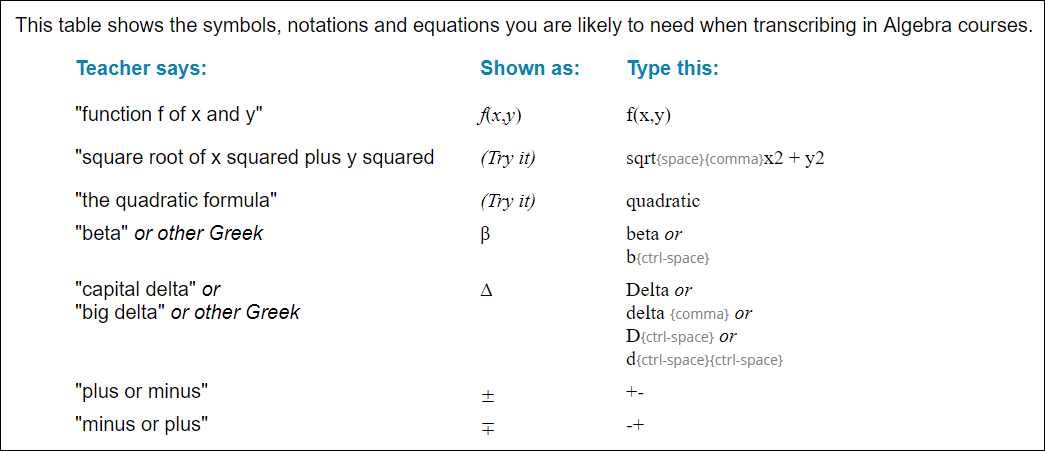# Math/Science Tutorial

1. What is the Math/Science Tutorial?
2. Will the Tutorial prepare me for transcribing math and science classes?
3. How do I access the Math/Science Tutorial?
4. What will I learn in the Math/Science Tutorial?
5. Where can I get more practice?

####What is the Math/Science Tutorial?

The tutorial is just that: a self-paced tutorial that explains how to use TypeWell's Math Mode, which is a separate dictionary mode in the TypeWell transcriber program. The tutorial is not a class or a course. It does not include any interactive drills. However, it does include instructions and examples, so that you can practice each demonstrated skill.

For example:It's up to the individual transcriber to practice what the tutorial teaches, by opening up the TypeWell Transcriber program and drilling the keystrokes that are demonstrated in the tutorial.

To prepare for a transcribing assignment that involves math or science, a transcriber should first study the sections of the tutorial that cover that particular branch of math or science. The transcriber should then practice those keystrokes, preferably with some recorded lectures online before the assignment begins.

####Will the Tutorial prepare me for transcribing math and science classes?

Not unless you put in significant practice ahead of time. The tutorial will teach you how to type symbols, expressions, and notations commonly encountered in math and science classes. This provides a fast way for transcribers to display math and science terms, quickly and correctly.

However, transcribing in such classes is still difficult because of the unique style of communication used to convey math and scientific concepts. Thus, beginning transcribers should not transcribe math and science classes.

We strongly recommend that transcribers should first work for at least one school term in non-math/science classes, to stabilize their basic transcribing skills, before learning the advanced skills taught in the tutorial. Then, after reviewing the tutorial, transcribers should practice with recorded online lectures (similar to the lectures they will be transcribing in real life) to prepare for real-time assignments.

At the bottom of this article, we have included links to several good websites that offer free recorded math/science lectures.

####How do I access the Math/Science Tutorial?

The Math/Science Tutorial is installed automatically with the TypeWell Transcriber software (versions 5 and higher) and is stored on the transcriber's local hard drive. To access the tutorial, open the TypeWell Transcriber program, click on the **Math **menu, then click Math Tutorial.

Click the chapter links along the top of the page to view each chapter of the tutorial.

####What will I learn in the Math/Science Tutorial?

Chapter 1 of the tutorial covers the "Basics", including how to get into and out of TypeWell's Math Mode, how Math Mode expands your abbreviations, and the principles of spacing and formatting in Math Mode.

Chapter 2 teaches the basic and advanced arithmetic symbols available in TypeWell Math Mode:

• Multiplication and Division
• Fractions (including fractions with letters or symbols, and the fraction template for fractions that require multiple lines)
• Long Division
• Superscripts and Subscripts (including number superscripts in polynomials, alphabet and multiple-term superscripts, special abbreviations for squared and cubed, two-level superscripts and subscripts, and subscripts for Chemistry and other sciences)
• Square Roots (including the square root template)
• Cube Roots and Other Roots
• Equality/Inequality Conditions

Chapter 3 teaches how to transcribe Greek letters and other symbols:

• Greek Alphabet
• Typing Letters or Numbers Before or Immediately After Symbols
• Using Symbols in Super/Subscript Position

Chapter 4 teaches how to transcribe symbols, characters, and notations used in all different branches of math and science:

Algebra Group Theory Physics
Arrows Integrals Real Numbers
Calculus Limits Sets
Chemistry Logic Statistics
Foreign Matrices Symbol Smarts
Geometry Miscellaneous Trigonometry

**Chapter 5 **explains how the Math PAL (Personal Abbreviation List) works.

####Where can I get more practice?

The tutorial provides instructions for displaying math and science terms in the TypeWell Transcriber program. Transcribers must then practice these skills in order to use them quickly and efficiently.
For basic, slower-paced lectures, we recommend the following:

For more advanced lectures, we recommend searching for specific subjects or branches of math and science lectures on:

Visit the TypeWell Learning Center for additional resources, including the Math Mode Basics series which provides hands-on practice to help transcribers improve their skills.